# Olympiad Test: Exponents And Powers -1

## 20 Questions MCQ Test Mathematical Olympiad Class 8 | Olympiad Test: Exponents And Powers -1

Description
Attempt Olympiad Test: Exponents And Powers -1 | 20 questions in 40 minutes | Mock test for Class 8 preparation | Free important questions MCQ to study Mathematical Olympiad Class 8 for Class 8 Exam | Download free PDF with solutions
QUESTION: 1

### What is the value of [(5- 1 × 3-1 ) -1 ÷ 6 - 1 ] ?

Solution: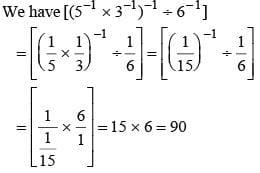QUESTION: 2

### ​What is the value of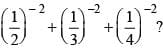Solution: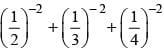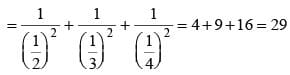QUESTION: 3

### What is the value of p for which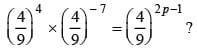Solution: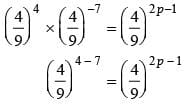2p – 1 = - 3
⇒ 2p = - 2
⇒ p = -1

QUESTION: 4

If 52x + 1 ÷ 25 = 125. What is the value of x?

Solution: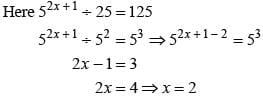QUESTION: 5

By what number should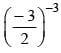be divided so that the quotient is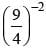?

Solution:

Let the number be x.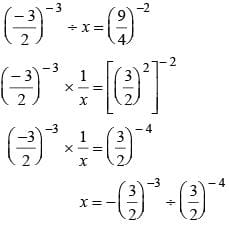x = -3/2

QUESTION: 6

What is the value of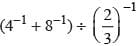?

Solution:

We have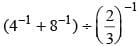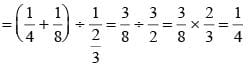QUESTION: 7

What is the value of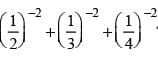?

Solution:
QUESTION: 8

By what number should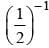be multiplied so that the product is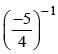?

Solution:

Let the number be x.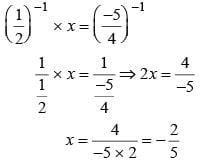QUESTION: 9

By what number should (-6)-1 be multiplied so that the product becomes 9-1?

Solution:

(- 6)-1 × x = 9-1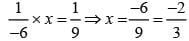QUESTION: 10

What is the standard form of 0.00000000837?

Solution:

We have 0.00000000837 =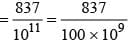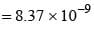QUESTION: 11

What is the value of x for which.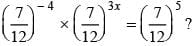Solution:
QUESTION: 12

The value of (3-1 + 4-1)-1 ÷ 5-1 =?

Solution:

Here (3-1 + 4-1)-1 ÷ 5-1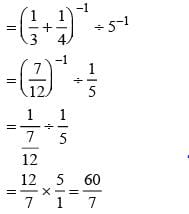QUESTION: 13

If (23x – 1 + 10) ÷ 7 = 6 then what is the value of x?

Solution:

Here (23x - 1 + 10) ÷ 7 = 6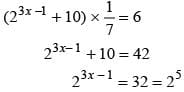On comparing the powers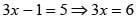x = 2

QUESTION: 14

What is the usual  form of 0.000467 × 104 ?

Solution:

0.000467 × 104.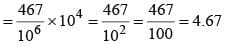QUESTION: 15

If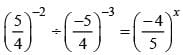, Find x?

Solution: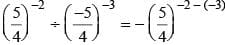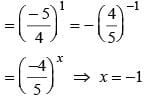QUESTION: 16

​What will be the value of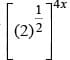, where x = - 0.5?

Solution: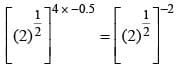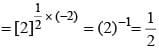QUESTION: 17

Express 1 micron in standard form.​

Solution: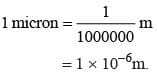QUESTION: 18

​The value of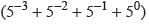is :

Solution: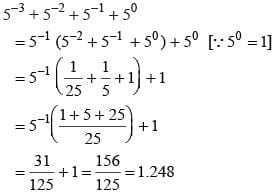QUESTION: 19

If 52p + 5p + 50 = 651, then p =

Solution: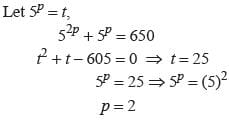QUESTION: 20

If then x =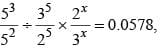then x =

Solution: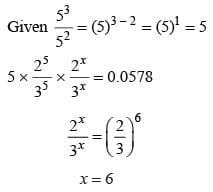Use Code STAYHOME200 and get INR 200 additional OFF Use Coupon Code